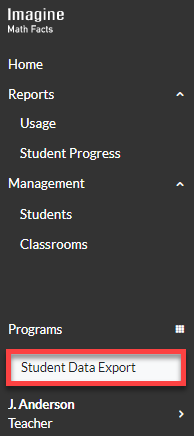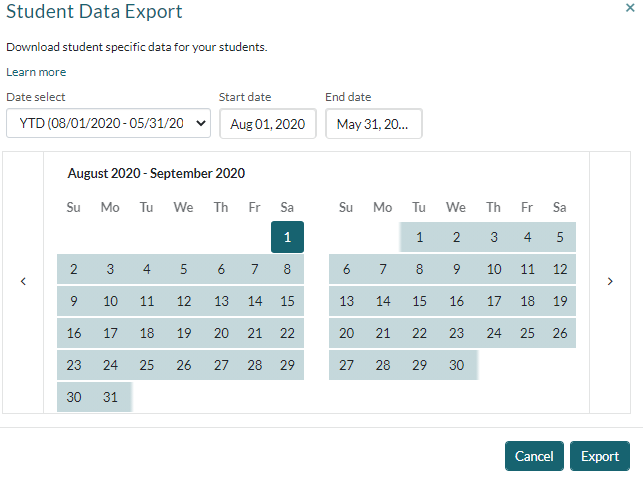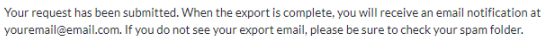# Exporting student data from Imagine Math Facts

As an Administrator or Educator, you can export all student report data for your organization as a CSV-formatted export file. When you export report data, your export file includes information such as student number, name, grade level, district, school, usage data, Pre and Post-Test scores and completion dates, and the number of math facts the student has encountered and passed since the beginning of the school year. (For more details about each column in the export file, see What data is included in the export file.)

When you export student data, you can choose the date range for your export file. You can only export data for previous daysyou can't export report data for the current day. Depending on the size of your class or organization and the amount of data you have, your export file may take up to 20 minutes to generate. Once your export file has been generated, you’ll receive an email with a link to download the export file.

To export student report data from Imagine Math Facts

1. Click Student Data Export in the navigation pane.2. Choose YTD or Custom to specify the date range for your export file. If you choose Custom, specify the Start date and End date.3. Click Export. You'll see this confirmation message:## What data is included in the export file

Field Description
First Name The student's first name in Imagine Math Facts.
Last Name The student's last name in Imagine Math Facts.
student_information_number

A unique identification number (typically their SIS identification number) assigned to the student when the student is rostered. This number identifies the student in Imagine Math Facts.

alt_student_information_number

A secondary student identification number in Imagine Math Facts (optional).

Note

This field is optional. If the Alternate Student Number has not been entered in Imagine Math Facts, this column will be empty in the export file.

is_active Identifies whether or not a student is currently active in Imagine Math Facts. If the student is active, the column displays "True"; if the student is inactive, the column displays "False."
is_ell Identifies whether or not a student is an English Language Learner (ELL). If the student is an ELL, the column displays "True"; if the student isn't an ELL, the column displays "False."
is_iep Identifies whether the student has an Individualized Education Plan. If the student has an IEP, the column displays "True"; if the student doesn't have an IEP, the column displays "False."
district The school district that the student is assigned to.
school The school that the student is assigned to.
classroom The classroom(s) that the student is assigned to. If a student is assigned to multiple classroom, each classroom name is listed in the column, separated by semi-colons.
teachers The teacher(s) that the student is assigned to. If a student is assigned to multiple teachers, each teacher's name is listed in the column, separated by semi-colons.
total_fact_time The total amount of time that students used Imagine Math Facts, including School and Home Time.
school_fact_time The amount of time that students used Imagine Math Facts during school hours (between 7 am–4 pm local time, Monday– Friday).
evening_fact_time The amount of time that students used Imagine Math Facts outside of school hours (between 4 pm–7 am local time, Monday–Friday, and all day Saturday–Sunday).
addition_pre_test_date The date the student completed the Addition Pre-test. If this column is empty, the student has not yet completed the Addition Pre-test.
addition_pretest_score The student's Addition Pre-test score. If this column is empty, the student has not yet completed the Addition Pre-test.
addition_posttest_date The date the student completed the Addition Post-test. If this column is empty, the student has not yet completed the Addition Post-test.
addition_posttest_score The student's Addition Post-test score. If this column is empty, the student has not yet completed the Addition Post-test.
addition_facts_encountered The number of Addition facts that the student attempted, whether passed, failed, or retaken.
addition_total_fact_time The total amount of time the student spent completing Addition facts, including School and Home Time.

The amount of time that students used Imagine Math Facts during school hours (between 7 am–4 pm local time, Monday– Friday) to complete Addition facts.

The amount of time that students used Imagine Math Facts outside of school hours (between 4 pm–7 am local time, Monday–Friday, and all day Saturday–Sunday) to complete Addition facts.

addition_estimated_time_remaining The estimated time remaining before the student can take the Addition Post-test.
subtraction_pre_test_date

The date the student completed the Subtraction Pre-test. If this column is empty, the student has not yet completed the Subtraction Pre-test.

subtraction_pretest_score The student's Subtraction Pre-test score. If this column is empty, the student has not yet completed the Subtraction Pre-test.
subtraction_posttest_date

The date the student completed the Subtraction Post-test. If this column is empty, the student has not yet completed the Subtraction Post-test.

subtraction_posttest_score The student's Subtraction Post-test score. If this column is empty, the student has not yet completed the Subtraction Post-test.
subtraction_facts_passed The number of Subtraction facts that the student answered correctly.
subtraction_facts_encountered The number of Subtraction facts that the student attempted, whether passed, failed, or retaken.
subtraction_total_fact_time The total amount of time the student spent completing Subtraction facts, including School and Home Time.
subtraction_school_fact_time The amount of time that students used Imagine Math Facts during school hours (between 7 am–4 pm local time, Monday– Friday) to complete Subtraction facts.
subtraction_evening_fact_time

The amount of time that students used Imagine Math Facts outside of school hours (between 4 pm–7 am local time, Monday–Friday, and all day Saturday–Sunday) to complete Subtraction facts.

subtraction_estimated_time_remaining The estimated time remaining before the student can take the Subtraction Post-test.
multiplication_pre_test_date The date the student completed the Multiplication Pre-test. If this column is empty, the student has not yet completed the Multiplication Pre-test.
multiplication_pretest_score

The student's Multiplication Pre-test score. If this column is empty, the student has not yet completed the Multiplication Pre-test.

multiplication_posttest_date

The date the student completed the Multiplication Post-test. If this column is empty, the student has not yet completed the Multiplication Post-test.

multiplication_posttest_score The student's Multiplication Post-test score. If this column is empty, the student has not yet completed the Multiplication Post-test.
multiplication_facts_passed The number of Multiplication facts that the student answered correctly.
multiplication_facts_encountered The number of Multiplication facts that the student attempted, whether passed, failed, or retaken.
multiplication_total_fact_time The total amount of time the student spent completing Multiplication facts, including School and Home Time.
multiplication_school_fact_time The amount of time that students used Imagine Math Facts during school hours (between 7 am–4 pm local time, Monday– Friday) to complete Multiplication facts.
multiplication_evening_fact_time

The amount of time that students used Imagine Math Facts outside of school hours (between 4 pm–7 am local time, Monday–Friday, and all day Saturday–Sunday) to complete Multiplication facts.

multiplication_estimated_time_remaining The estimated time remaining before the student can take the Multiplication Post-test.
division_pre_test_date

The date the student completed the Division Pre-test. If this column is empty, the student has not yet completed the Division Pre-test.

division_pretest_score The student's Division Pre-test score. If this column is empty, the student has not yet completed the Division Pre-test.
division_posttest_date

The date the student completed the Division Post-test. If this column is empty, the student has not yet completed the Division Post-test.

division_posttest_score The student's Division Post-test score. If this column is empty, the student has not yet completed the Division Post-test.
division_facts_passed The number of Division facts that the student answered correctly.
division_facts_encountered The number of Division facts that the student attempted, whether passed, failed, or retaken.
division_total_fact_time The total amount of time the student spent completing Division facts, including School and Home Time.
division_school_fact_time The amount of time that students used Imagine Math Facts during school hours (between 7 am–4 pm local time, Monday– Friday) to complete Division facts.
division_evening_fact_time

The amount of time that students used Imagine Math Facts outside of school hours (between 4 pm–7 am local time, Monday–Friday, and all day Saturday–Sunday) to complete Division facts.

division_estimated_time_remaining The estimated time remaining before the student can take the Division Post-test.